# Geometry functions

The following functions allow you to create, compare, measure, and analyze geometries. The spatial reference of all geometries either input or created by any of these functions must match the spatial reference of the profile's context, which controls the execution of the expression.

## Angle

This function has 2 signatures:

#### Angle(pointA, pointB) -> Number

Since version 1.7

Function bundle: Geometry

Returns the arithmetic angle of a line between two points in degrees (0 - 360). The angle is measured in a counter-clockwise direction relative to east. For example, an angle of 90 degrees points due north.

Only the x-y plane is considered for the measurement. Any z-coordinates are ignored. Point features can be used instead of any or both Point geometries. If the points are identical, then an angle of 0 degrees is returned.

Parameters

• pointA: Point | Feature - The first Point or Feature used to calculate the angle.
• pointB: Point | Feature - The second Point or Feature used to calculate the angle.

Return value: Number##### Example

Returns the angle from a Point to a Feature, in degrees

Use dark colors for code blocksCopy
``````var pointA = Point({ "x":976259, "y":8066511, "spatialReference": { "wkid": 3857 } });
Angle(pointA, \$feature)``````

#### Angle(pointA, pointB, pointC) -> Number

Since version 1.7

Function bundle: Geometry

Returns the arithmetic angle of a line between three points in degrees (0 - 360). The angle is measured around `pointB` in a counter-clockwise direction, from `pointA` to `pointC`.

Only the x-y plane is considered for the measurement. Any z-coordinates are ignored. Point features can be used instead of either or all Point geometries. If the points are identical, then an angle of 0 or 180 degrees is returned (depending internal arithmetic).

Parameters

• pointA: Point | Feature - The first Point or Feature used to calculate the angle.
• pointB: Point | Feature - The second Point or Feature used to calculate the angle.
• pointC: Point | Feature - The third Point or Feature used to calculate the angle.

Return value: Number##### Example

Returns the angle between two points around the feature, in degrees

Use dark colors for code blocksCopy
``````var pointA = Point({ "x":976259, "y":8066511, "spatialReference": { "wkid": 3857 } });
var pointC = Point({ "x":308654, "y":9005421, "spatialReference": { "wkid": 3857 } });
Angle(pointA, \$feature, pointC)``````

## Area

#### Area(polygon, unit?) -> Number

Since version 1.7

Function bundle: Geometry

Returns the area of the input geometry or Feature in the given units. This is a planar measurement using Cartesian mathematics.

Feature geometries in the visualization and labeling profiles are generalized according to the view's scale resolution to improve drawing performance. Therefore, using a feature's geometry (i.e. `\$feature`) as input to any geometry function in these contexts will return different results at each scale level. Other profiles, such as popup, provide the full resolution geometry.

Parameters

• polygon: Polygon | Feature | Array<Point> - The Polygon or Feature for which to calculate the planar area.
• unit (Optional): Text | Number - Measurement unit of the return value.
Possible values: `acres` | `square-feet` | `hectares` | `square-kilometers` | `square-miles` | `square-nautical-miles` | `square-meters` | `square-yards`

Return value: Number

##### Example

Returns the area of the feature in square meters

Use dark colors for code blocksCopy
``Area(\$feature, 'square-meters')``

## AreaGeodetic

#### AreaGeodetic(polygon, unit?) -> Number

Since version 1.7

Function bundle: Geometry

Returns the geodetic area of the input geometry or Feature in the given units. This is more reliable measurement of area than `Area()` because it takes into account the Earth's curvature. Support is limited to geometries with a Web Mercator (wkid 3857) or a WGS 84 (wkid 4326) spatial reference.

Feature geometries in the visualization and labeling profiles are generalized according to the view's scale resolution to improve drawing performance. Therefore, using a feature's geometry (i.e. `\$feature`) as input to any geometry function in these contexts will return different results at each scale level. Other profiles, such as popup, provide the full resolution geometry.

Parameters

• polygon: Polygon | Feature | Array<Point> - The Polygon or Feature for which to calculate the geodetic area.
• unit (Optional): Text | Number - Measurement unit of the return value.
Possible values: `acres` | `square-feet` | `hectares` | `square-kilometers` | `square-miles` | `square-nautical-miles` | `square-meters` | `square-yards`

Return value: Number

##### Example

Returns the geodetic area of the feature in square meters

Use dark colors for code blocksCopy
``AreaGeodetic(\$feature, 'square-meters')``

## Bearing

This function has 2 signatures:

#### Bearing(pointA, pointB) -> Number

Since version 1.7

Function bundle: Geometry

Returns the geographic angle of a line between two points in degrees (0 - 360). The bearing is measured in a clockwise direction relative to north. For example, a bearing of 225 degrees represents a southwest orientation.

Only the x-y plane is considered for the measurement. Any z-coordinates are ignored. Point features can be used instead of either or both Point geometries. If the points are identical, then an angle of 0 is returned.

Parameters

• pointA: Point | Feature - The first point used to calculate the bearing.
• pointB: Point | Feature - The second point used to calculate the bearing.

Return value: Number##### Example

Returns the bearing from a point to the feature, in degrees

Use dark colors for code blocksCopy
``````var pointA = Point({ "x":976259, "y":8066511, "spatialReference": { "wkid": 3857 } });
Bearing(pointA,\$feature)``````

#### Bearing(pointA, pointB, pointC) -> Number

Since version 1.7

Function bundle: Geometry

Returns the geographic angle of a line between three points in degrees (0 - 360). The bearing is measured around `pointB` in a clockwise direction, from `pointA` to `pointC`.

Only the x-y plane is considered for the measurement. Any z-coordinates are ignored. Point features can be used instead of any or all Point geometries. If the points are identical, then an angle of 0 or 180 degrees is returned (depending internal arithmetic).

Parameters

• pointA: Point | Feature - The first point used to calculate the bearing.
• pointB: Point | Feature - The second point used to calculate the bearing.
• pointC: Point | Feature - The third point used to calculate the bearing.

Return value: Number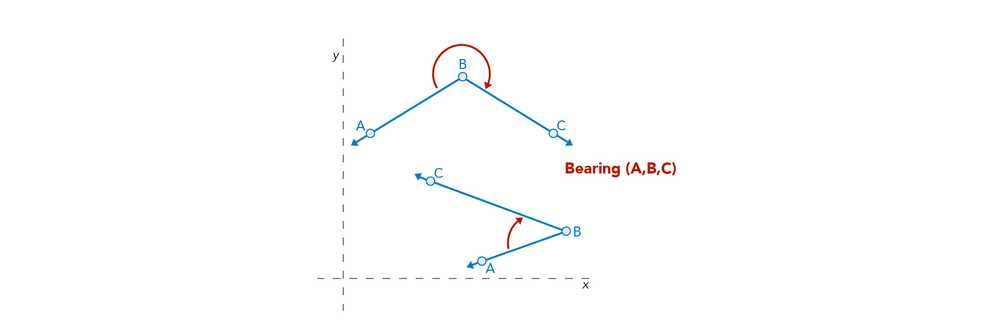##### Example

Returns the bearing between two points around the feature, in degrees

Use dark colors for code blocksCopy
``````var pointA = Point({ "x":976259, "y":8066511, "spatialReference": { "wkid": 3857 } });
var pointC = Point({ "x":308654, "y":9005421, "spatialReference": { "wkid": 3857 } });
Bearing(pointA,\$feature,pointC)``````

## Buffer

#### Buffer(inputGeometry, distance, unit?) -> Polygon

Since version 1.3

Function bundle: Geometry

Returns the planar (or Euclidean) buffer at a specified distance around the input geometry. This is a planar measurement using Cartesian mathematics.

Feature geometries in the visualization and labeling profiles are generalized according to the view's scale resolution to improve drawing performance. Therefore, using a feature's geometry (i.e. `\$feature`) as input to any geometry function in these contexts will return different results at each scale level. Other profiles, such as popup, provide the full resolution geometry.

Parameters

• inputGeometry: Geometry | Feature - The geometry to buffer.
• distance: Number - The distance to buffer from the geometry.
• unit (Optional): Text | Number - Measurement unit of the buffer `distance`. For the visualization, labeling, and popup profiles, the default unit is the map's spatial reference. In other profiles, like field calculation, the default is based on the spatial reference of the data.
Possible values: `feet` | `kilometers` | `miles` | `nautical-miles` | `meters` | `yards`

Return value: Polygon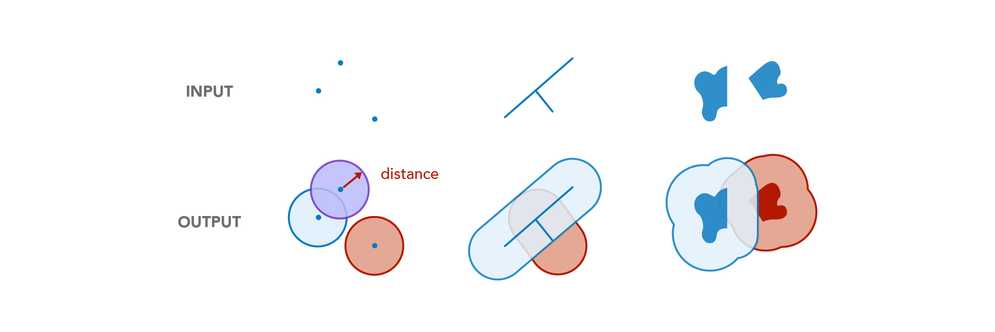##### Example

Returns a polygon representing a 1/2-mile buffer around the input geometry

Use dark colors for code blocksCopy
``Buffer(\$feature, 0.5, 'miles')``

## BufferGeodetic

#### BufferGeodetic(inputGeometry, distance, unit?) -> Polygon

Since version 1.3

Function bundle: Geometry

Returns the geodetic buffer at a specified distance around the input geometry. This is a geodetic measurement, which calculates distances on an ellipsoid. Support is limited to geometries with a Web Mercator (wkid 3857) or a WGS 84 (wkid 4326) spatial reference.

Feature geometries in the visualization and labeling profiles are generalized according to the view's scale resolution to improve drawing performance. Therefore, using a feature's geometry (i.e. `\$feature`) as input to any geometry function in these contexts will return different results at each scale level. Other profiles, such as popup, provide the full resolution geometry.

Parameters

• inputGeometry: Geometry | Feature - The geometry to buffer.
• distance: Number - The distance to buffer from the geometry.
• unit (Optional): Text | Number - Measurement unit of the buffer `distance`. For the visualization, labeling, and popup profiles, the default unit is the map's spatial reference. In other profiles, like field calculation, the default is based on the spatial reference of the data.
Possible values: `feet` | `kilometers` | `miles` | `nautical-miles` | `meters` | `yards`

Return value: Polygon

##### Example

Returns a polygon representing a 1/2-mile buffer around the input geometry

Use dark colors for code blocksCopy
``BufferGeodetic(\$feature, 0.5, 'miles')``

## Centroid

#### Centroid(polygon) -> Point

Since version 1.7

Function bundle: Geometry

Returns the centroid of the input geometry.

Parameter

Return value: Point

##### Examples

Returns the centroid of the given polygon

Use dark colors for code blocksCopy
``Centroid(\$feature)``

Returns the centroid of the given polygon ring

Use dark colors for code blocksCopy
``````var ringPoints = Geometry(\$feature).rings;
Centroid(ringPoints);``````

## Clip

#### Clip(inputGeometry, envelope) -> Geometry

Since version 1.3

Function bundle: Geometry

Calculates the clipped geometry from a target geometry by an envelope.

Feature geometries in the visualization and labeling profiles are generalized according to the view's scale resolution to improve drawing performance. Therefore, using a feature's geometry (i.e. `\$feature`) as input to any geometry function in these contexts will return different results at each scale level. Other profiles, such as popup, provide the full resolution geometry.

Parameters

• inputGeometry: Geometry | Feature - The geometry to be clipped.
• envelope: Extent - The envelope used to clip the `geometry`.

Return value: Geometry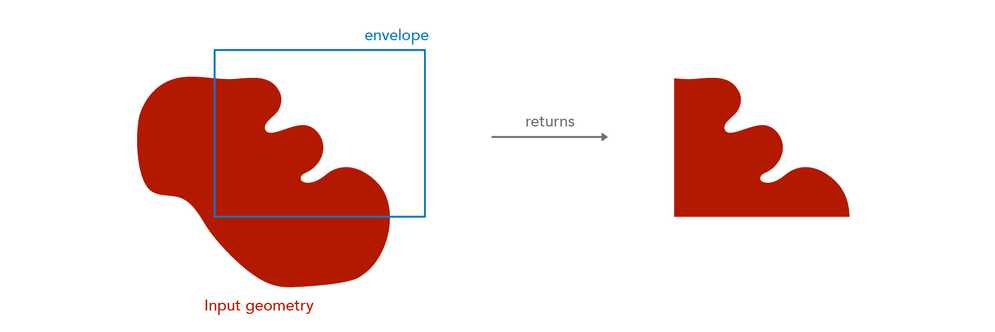##### Example

Returns the area of the clipped geometry

Use dark colors for code blocksCopy
``````var envelope = Extent({ ... });
Area(Clip(\$feature, envelope), 'square-miles');``````

## Contains

This function has 2 signatures:

#### Contains(containerGeometry, insideGeometry) -> Boolean

Since version 1.7

Function bundle: Geometry

Indicates if one geometry contains another geometry. In the graphic below, the red highlight indicates the scenarios where the function will return `true`.

Be aware that using `\$feature` as input to this function will yield results only as precise as the view's scale resolution. Therefore values returned from expressions using this function may change after zooming between scales.

Parameters

• containerGeometry: Geometry | Feature - The geometry that is tested for the 'contains' relationship to `insideGeometry`. Think of this geometry as the potential 'container' of the `insideGeometry`.
• insideGeometry: Geometry | Feature - The geometry that is tested for the 'within' relationship to the `containerGeometry`.

Return value: Boolean##### Example

Returns true if the feature is contained within the given polygon

Use dark colors for code blocksCopy
``````var container = Polygon({ ... });
Contains(containerGeometry, \$feature);``````

#### Contains(containerGeometry, insideFeatures) -> FeatureSet

Since version 1.7

Function bundle: Geometry

Returns features from a FeatureSet that are contained within the input geometry. In the graphic below, the red highlight illustrates the spatial relationships where the function will return features.

Be aware that using `\$feature` as input to this function will yield results only as precise as the view's scale resolution. Therefore values returned from expressions using this function may change after zooming between scales.

Parameters

• containerGeometry: Geometry | Feature - The geometry that is tested for the 'contains' relationship to `insideFeatures`. Think of this geometry as the potential 'container' of the `insideFeatures`.
• insideFeatures: FeatureSet - The FeatureSet that is tested for the 'within' relationship to the `containerGeometry`.

Return value: FeatureSet##### Example

Returns the number of features that are within the given polygon

Use dark colors for code blocksCopy
``````var parcels = FeatureSetByName(\$map, 'parcels')
var projectArea = \$feature;
Count(Contains(projectArea, parcels));``````

## ConvertDirection

#### ConvertDirection(input, inputSpec, outputSpec) -> Array<Number | Text> | Number | Text

Since version 1.13

Function bundle: Geometry

Angles can have several interpretations and can be represented as a number, a text, or a well formed array. This function takes one input representation and converts it to another.

The input value is described by a dictionary that specified the type of angle and the type of direction. Examples:

inputangle typedirection type
[12,34,56]dmsnorth, south, polar
'12 34 56'dmsnorth, south, polar

If the `angleType` and `directionType` are not appropriate for the input, then the conversion will fail.

The desired output value is as well described by a dictionary that specidies output type, angle type, direction type, and an optional format for text ouput.

If the output type is `value`:

• an array will be returned for angle type `dms` or for direction type `quadrant`
• a number will be returned for all the other cases

If the output type is `text`, then default padding and delimeters will be used unless the optional`format` property is provided.
`format` controls order, spacing, padding, and delimeters in the output text.
Strings of format specifier characters before a decimal point indicate minimum padding (e.g. `DDD -> 000`).
Strings of format characters after a decimal point indicate precision (e.g. `D.DD -> 0.00`).

Supported `format` characters:

CodeMeaning
`D`Decimal Degrees
`R`Radians
`G`Gradians
`d`DMS Degrees
`m`DMS Minutes
`s`DMS Seconds
`P`Long Meridian (e.g. `North` vs. `South`)
`p`Short Meridian (e.g. `N` vs. `S`)
`B`Long direction (e.g. `East` vs. `West`)
`b`Short direction (e.g. `E` vs. `W`)
`[ ]`Escape characters

For `dms` formatting, if the `s` is not used then `m` will round to the nearest minute. Similarly, if `m` is not used then `d` will round.

Parameters

• input: Array<Number | Text> | Number | Text - A raw representation of the bearing. The type of `input` and the values of the `inputSpec` dictate how the input is parsed.

• inputSpec: Dictionary - Contains information about how to interpret input.

• angleType: Text - Describes the input angle unit. Supported Values: `DEGREES`, `DMS`, `RADIANS`, `GONS`, `GRADIANS`
• directionType: Text - Describes the input bearing's meridian and direction. Supported Values: `NORTH`, `SOUTH`, `POLAR`, `QUADRANT`
• outputSpec: Dictionary - Contains information about how to format the output.

• outputType: Text - Controls output type. Supported Values: `value`, `text`
• angleType: Text - Describes the output angle unit. Supported Values: `DEGREES`, `DMS`, `RADIANS`, `GONS`, `GRADIANS`
• directionType: Text - Describes the output bearing's meridian and direction. Supported Values: `NORTH`, `SOUTH`, `POLAR`, `QUADRANT`
• format (Optional): Text - Controls text formatting. Only applicable if `outputType` is `text`.

Return value: Array<Number | Text> | Number | Text

##### Examples

Examples where the `outputType` is `value`.

Use dark colors for code blocksCopy
``````ConvertDirection( 30, {directionType:'North', angleType: 'Degrees'}, {directionType:'Quadrant', angleType: 'DMS', outputType: 'value'})
// returns ['N', 30, 0, 0, 'E']

ConvertDirection( 25.99, {directionType:'North', angleType : 'Gradians'}, {directionType:'North', outputType: 'value', angleType : 'Gradians'})
// returns 25.99

ConvertDirection( 1, {directionType:'North', angleType: 'DEGREES'}, {directionType: 'Quadrant', angleType: 'Degrees', outputType: 'value'})
// returns ['N',1,'E']

ConvertDirection( 0.9, {directionType: 'North', angleType: 'degrees'}, {directionType:'North', angleType: 'gradians', outputType: 'value'})
// returns 1.0

ConvertDirection( 180.0, {directionType:'North', angleType: 'degrees'}, {directionType:'North', angleType: 'radians', outputType : 'value'})
// returns PI``````

Examples where `outputType` is `text`.

Use dark colors for code blocksCopy
``````ConvertDirection( 25.34, {directionType: 'North', angleType: 'DEGREES'}, {directionType:'North', outputType: 'text', format: 'DDDD.D'})
// returns '0025.3'

ConvertDirection( 25.34, {directionType: 'North', angleType: 'DEGREES'}, {directionType:'North', outputType: 'text', format: 'R'})
// returns '0'

ConvertDirection( 25.34, {directionType: 'North', angleType: 'DEGREES'}, {directionType:'North', outputType: 'text', format: '[DD.DD]'})
// returns 'DD.DD'

ConvertDirection( 25.34, {directionType:'North', angleType: 'DEGREES'}, {directionType:'quadrant', outputType: 'text', format: 'P B'})
// returns 'North East'

ConvertDirection( [001,01,59.99], {directionType:'North', angleType: 'DMS'}, {directionType:'North', angleType: 'DMS', outputType: 'text', format: 'dddA mm[B] ssC'})
// returns '001A 02B 00C'``````

## ConvexHull

#### ConvexHull(inputGeometry) -> Geometry

Since version 1.19

Function bundle: Geometry

Calculates the convex hull of a geometry. A convex hull is the smallest convex polygon that encloses a geometry. The hull is typically a polygon but can also be a polyline or a point in degenerate cases.

Be aware that using `\$feature` as input to this function will yield results only as precise as the view's scale resolution. Therefore values returned from expressions using this function may change after zooming between scales.

Parameter

• inputGeometry: Geometry | Feature - The point, line, or polygon geometry to be analyzed.

Return value: Geometry

##### Examples

Returns the number of vertices in the convex hull geometry of the current feature's geometry

Use dark colors for code blocksCopy
``Count(ConvexHull(Geometry(\$feature)).Rings)``

Returns the convex hull of a geometry which has a concave region (which the convex hull will ignore)

Use dark colors for code blocksCopy
``````var pacman_like_shape = Polygon({
"rings": [[[1, 2], [2, 0], [1, -2], [-1, -2], [-2, -1], [-1, -1.5], [0, -1.5], [-2, 1], [-1, 2]]],
"spatialReference": { "wkid": 3857 }
});
return ConvexHull(pacman_like_shape).rings;
// Returns the geometry [[1,2],[2,0],[1,-2],[-1,-2],[-2,-1],[-2,1],[-1,2],[1,2]]``````

## Crosses

#### Crosses(geometry1, geometry2) -> Boolean

Since version 1.3

Function bundle: Geometry

Indicates if one geometry crosses another geometry. In the graphic below, the red highlight indicates the scenarios where the function will return `true`.

Feature geometries in the visualization and labeling profiles are generalized according to the view's scale resolution to improve drawing performance. Therefore, using a feature's geometry (i.e. `\$feature`) as input to any geometry function in these contexts will return different results at each scale level. Other profiles, such as popup, provide the full resolution geometry.

Parameters

Return value: Boolean##### Example

Returns true if the feature crosses the given polygon

Use dark colors for code blocksCopy
``````var geom2 = Polygon({ ... });
Crosses(\$feature, geom2);``````

## Cut

#### Cut(polylineOrPolygon, cutter) -> Array<Geometry>

Since version 1.3

Function bundle: Geometry

Splits the input Polyline or Polygon where it crosses a cutting Polyline. For Polylines, all resulting left cuts are grouped together in the first Geometry. Right cuts and coincident cuts are grouped in the second Geometry. Each undefined cut, along with any uncut parts, are output as separate Polylines.

For Polygons, all resulting left cuts are grouped in the first Polygon, all right cuts are grouped in the second Polygon, and each undefined cut, along with any left-over parts after cutting, are output as a separate Polygon. If no cuts are returned then the array will be empty. An undefined cut will only be produced if a left cut or right cut was produced and there was a part left over after cutting, or a cut is bounded to the left and right of the cutter.

Feature geometries in the visualization and labeling profiles are generalized according to the view's scale resolution to improve drawing performance. Therefore, using a feature's geometry (i.e. `\$feature`) as input to any geometry function in these contexts will return different results at each scale level. Other profiles, such as popup, provide the full resolution geometry.

Parameters

Return value: Array<Geometry>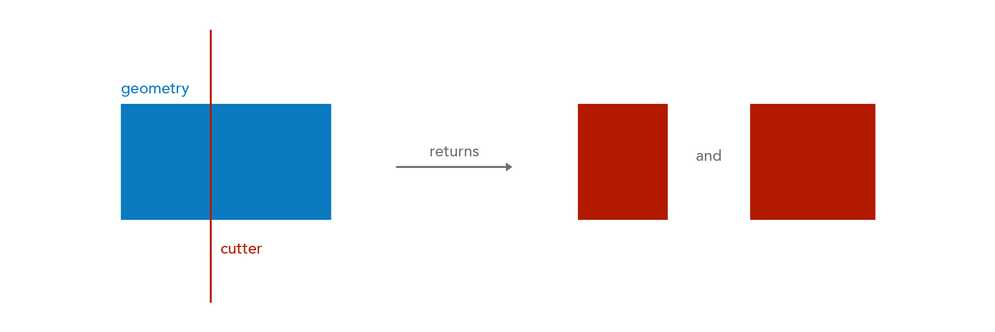##### Example

Cuts the feature's geometry with the given polyline

Use dark colors for code blocksCopy
``````var cutter = Polyline({ ... });
Cut(\$feature, cutter));``````

## Densify

#### Densify(inputGeometry, maxSegmentLength, unit?) -> Geometry

Since version 1.11

Function bundle: Geometry

Densifies geometries by inserting vertices to create segments no longer than the specified interval.

Feature geometries in the visualization and labeling profiles are generalized according to the view's scale resolution to improve drawing performance. Therefore, using a feature's geometry (i.e. `\$feature`) as input to any geometry function in these contexts will return different results at each scale level. Other profiles, such as popup, provide the full resolution geometry.

Parameters

• inputGeometry: Geometry | Feature - The input geometry to be densified.
• maxSegmentLength: Number - The maximum segment length allowed. Must be a positive value.
• unit (Optional): Text | Number - Measurement unit for maxSegmentLength. Defaults to the units of the input geometry. For the visualization, labeling, and popup profiles, the default unit is the map's spatial reference. In other profiles, like field calculation, the default is based on the spatial reference of the data.
Possible values: `feet` | `kilometers` | `miles` | `nautical-miles` | `meters` | `yards`

Return value: Geometry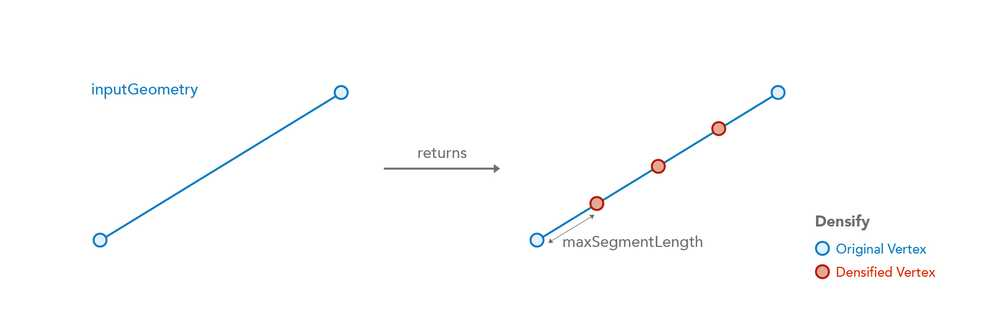##### Example

Returns the densified geometry with a maximum segment length of 10 m

Use dark colors for code blocksCopy
``````var maxLength = 10;
Densify(\$feature, maxLength, 'meters');``````

## DensifyGeodetic

#### DensifyGeodetic(inputGeometry, maxSegmentLength, unit?) -> Geometry

Since version 1.11

Function bundle: Geometry

Creates a geodesic between each of the input geometry's vertices and densifies the output geometry with additional vertices to create segments no longer than the specified interval.

Be aware that using `\$feature` as input to this function will yield results only as precise as the view's scale resolution. Therefore values returned from expressions using this function may change after zooming between scales.

Parameters

• inputGeometry: Geometry | Feature - The input geometry to be densified.
• maxSegmentLength: Number - The maximum segment length allowed. Must be a positive value.
• unit (Optional): Text | Number - Measurement unit for maxSegmentLength. Defaults to the units of the input geometry. For the visualization, labeling, and popup profiles, the default unit is the map's spatial reference. In other profiles, like field calculation, the default is based on the spatial reference of the data.
Possible values: `feet` | `kilometers` | `miles` | `nautical-miles` | `meters` | `yards`

Return value: Geometry##### Example

Returns the densified geometry with a maximum segment length of 10000

Use dark colors for code blocksCopy
``DensifyGeodetic(\$feature, 10000, 'meters');``

## Difference

#### Difference(inputGeometry, subtractor) -> Geometry

Since version 1.3

Function bundle: Geometry

Performs the topological difference operation for the two geometries. The resultant geometry comes from `inputGeometry`, not the `subtractor`. The dimension of `subtractor` has to be equal to or greater than that of `inputGeometry`.

Feature geometries in the visualization and labeling profiles are generalized according to the view's scale resolution to improve drawing performance. Therefore, using a feature's geometry (i.e. `\$feature`) as input to any geometry function in these contexts will return different results at each scale level. Other profiles, such as popup, provide the full resolution geometry.

Parameters

• inputGeometry: Geometry | Feature - The input geometry from which to subtract.
• subtractor: Geometry | Feature - The geometry to subtract from `geometry`.

Return value: Geometry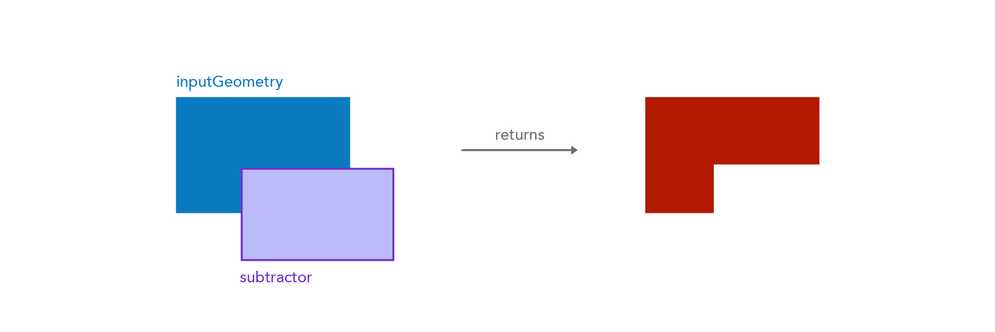##### Example

Subtracts the given polygon area from the feature.

Use dark colors for code blocksCopy
``````var subtractor = Polygon({ ... });
Difference(\$feature, subtractor);``````

## Disjoint

#### Disjoint(geometry1, geometry2) -> Boolean

Since version 1.3

Function bundle: Geometry

Indicates if one geometry is disjoint (doesn't intersect in any way) with another geometry. In the table below, the red highlight indicates that the function would return `true` with the specified geometries.

Feature geometries in the visualization and labeling profiles are generalized according to the view's scale resolution to improve drawing performance. Therefore, using a feature's geometry (i.e. `\$feature`) as input to any geometry function in these contexts will return different results at each scale level. Other profiles, such as popup, provide the full resolution geometry.

Parameters

• geometry1: Geometry | Feature - The base geometry that is tested for the 'disjoint' relationship to `geometry2`.
• geometry2: Geometry | Feature - The comparison geometry that is tested for the 'disjoint' relationship to `geometry1`.

Return value: Boolean##### Example

Returns true if the geometries don't intersect

Use dark colors for code blocksCopy
``````var geom2 = Polygon({ ... });
Disjoint(\$feature, geom2);``````

## Distance

#### Distance(geometry1, geometry2, unit?) -> Number

Since version 1.7

Function bundle: Geometry

Returns the planar distance between two geometries in the given units. This is a planar measurement using Cartesian mathematics.

Feature geometries in the visualization and labeling profiles are generalized according to the view's scale resolution to improve drawing performance. Therefore, using a feature's geometry (i.e. `\$feature`) as input to any geometry function in these contexts will return different results at each scale level. Other profiles, such as popup, provide the full resolution geometry.

Parameters

• geometry1: Geometry | Feature | Array<Point> - The geometry used to measure the distance from `geometry2`.
• geometry2: Geometry | Feature | Array<Point> - The geometry used to measure the distance from `geometry1`.
• unit (Optional): Text | Number - Measurement unit of the return value. For the visualization, labeling, and popup profiles, the default unit is the map's spatial reference. In other profiles, like field calculation, the default is based on the spatial reference of the data.
Possible values: `feet` | `kilometers` | `miles` | `nautical-miles` | `meters` | `yards`

Return value: Number

##### Example

Returns the distance between two geometries in meters

Use dark colors for code blocksCopy
``````var geom2 = Point({ ... });
Distance(\$feature, geom2, 'meters')``````

## DistanceGeodetic

#### DistanceGeodetic(point1, point2, units?) -> Number

Since version 1.8

Function bundle: Geometry

Calculates the shortest distance between two points along a great circle. Applies only to points with a Geographic Coordinate System (GCS) or the Web Mercator spatial reference. If the input points have a Projected Coordinate System (other than Web Mercator), you should use the `Distance()` function.

Feature geometries in the visualization and labeling profiles are generalized according to the view's scale resolution to improve drawing performance. Therefore, using a feature's geometry (i.e. `\$feature`) as input to any geometry function in these contexts will return different results at each scale level. Other profiles, such as popup, provide the full resolution geometry.

Parameters

• point1: Point | Feature - The point used to measure the distance from `point2`. This point must have a GCS or Web Mercator spatial reference.
• point2: Point | Feature - The point used to measure the distance from `point1`. This point must have a GCS or Web Mercator spatial reference.
• units (Optional): Text | Number - Measurement unit of the return value. For the visualization, labeling, and popup profiles, the default unit is the map's spatial reference. In other profiles, like field calculation, the default is based on the spatial reference of the data.
Possible values: `feet` | `kilometers` | `miles` | `nautical-miles` | `meters` | `yards`

Return value: Number

##### Example

Returns the distance from a bus in a stream layer to the central station in kilometers

Use dark colors for code blocksCopy
``````var unionStation = Point({"x": -118.15, "y": 33.80, "spatialReference": { "wkid": 3857 }});
distanceGeodetic(\$feature, unionStation, 'kilometers');``````

## EnvelopeIntersects

#### EnvelopeIntersects(geometry1, geometry2) -> Boolean

Since version 1.11

Function bundle: Geometry

Indicates if the envelope (or extent) of one geometry intersects the envelope of another geometry. In the graphic below, the red highlight indicates the scenarios where the function will return `true`.

Feature geometries in the visualization and labeling profiles are generalized according to the view's scale resolution to improve drawing performance. Therefore, using a feature's geometry (i.e. `\$feature`) as input to any geometry function in these contexts will return different results at each scale level. Other profiles, such as popup, provide the full resolution geometry.

Parameters

• geometry1: Geometry | Feature - The geometry that is tested for the intersects relationship to the other geometry.
• geometry2: Geometry | Feature - The geometry being intersected.

Return value: Boolean##### Example

Returns true if the geometries intersect

Use dark colors for code blocksCopy
``````var geom2 = Polygon({ ... });
EnvelopeIntersects(\$feature, geom2);``````

## Equals

#### Equals(geometry1, geometry2) -> Boolean

Since version 1.3

Function bundle: Geometry

Indicates if two geometries are equal, or geographically equivalent given the spatial reference and tolerance of the data. The two input geometries don't have to be clones to be considered equal.

Feature geometries in the visualization and labeling profiles are generalized according to the view's scale resolution to improve drawing performance. Therefore, using a feature's geometry (i.e. `\$feature`) as input to any geometry function in these contexts will return different results at each scale level. Other profiles, such as popup, provide the full resolution geometry.

Parameters

Return value: Boolean

##### Example

Returns true if the geometries are equal

Use dark colors for code blocksCopy
``````var geom2 = Point({ ... });
Equals(\$feature, geom2);``````

## Extent

This function has 3 signatures:

#### Extent(geometryDefinition) -> Extent

Function bundle: Geometry

Constructs an Extent object from a dictionary.

Parameter

• geometryDefinition: Dictionary - The properties from which to construct the Extent geometry object.

• xMax: Number - The upper bound, or largest possible x-coordinate of the geometry.

• xMin: Number - The lower bound, or smallest possible x-coordinate of the geometry.

• yMax: Number - The upper bound, or largest possible y-coordinate of the geometry.

• yMin: Number - The lower bound, or smallest possible y-coordinate of the geometry.

• mMax: Number - The upper bound, or largest possible m-value of the geometry. If setting this value, then you must set `hasM` to `true`.

• mMin: Number - The lower bound, or smallest possible m-value of the geometry. If setting this value, then you must set `hasM` to `true`.

• zMax: Number - The upper bound, or largest possible z-value of the geometry. If setting this value, then you must set `hasZ` to `true`.

• zMin: Number - The lower bound, or smallest possible z-value of the geometry. If setting this value, then you must set `hasZ` to `true`.

• hasM: Boolean - Indicates if the geometry has m-values.

• hasZ: Boolean - Indicates if the geometry has z-values.

• spatialReference: Dictionary - The spatial reference of the geometry.

• wkid: Number - The Well-known ID of the geographic or projected coordinate system that defines the reference for which to draw the geometry.

Return value: Extent

##### Example

Creates an Extent object

Use dark colors for code blocksCopy
``````Extent({
xMax: -95.34,
xMin: -97.06138,
yMax: 32.837,
yMin: 12.003,
hasM: false,
hasZ: false,
spatialReference: { wkid: 3857 }
});``````

#### Extent(inputGeometry) -> Extent

Function bundle: Geometry

Returns the extent of an input Feature, Polygon, Point, Polyline or Multipoint.

Feature geometries in the visualization and labeling profiles are generalized according to the view's scale resolution to improve drawing performance. Therefore, using a feature's geometry (i.e. `\$feature`) as input to any geometry function in these contexts will return different results at each scale level. Other profiles, such as popup, provide the full resolution geometry.

Parameter

• inputGeometry: Geometry | Feature - The Feature or Geometry from which to get an extent.

Return value: Extent

##### Example

Returns the extent of a feature

Use dark colors for code blocksCopy
``Extent(\$feature);``

#### Extent(jsonDefinition) -> Extent

Function bundle: Geometry

Constructs an Extent object from serialized JSON text. The JSON schema must follow the ArcGIS REST API JSON specification for Envelope objects.

Parameter

• jsonDefinition: Text - The JSON text from which to construct the extent object.

Return value: Extent

##### Example

Creates an Extent object from JSON

Use dark colors for code blocksCopy
``````var extentJSON = '{"xmin": -109.55, "ymin": 25.76, "xmax": -86.39, "ymax": 49.94, "spatialReference": { "wkid": 3857 }}';
Extent(extentJSON);``````

## Generalize

#### Generalize(inputGeometry, maxDeviation, removeDegenerateParts?, maxDeviationUnit?) -> Geometry

Since version 1.11

Function bundle: Geometry

Reduces the number of vertices in the input geometry based on a given deviation value. Point and Multipoint geometries are left unchanged. Envelopes are converted to Polygons and then generalized.

Feature geometries in the visualization and labeling profiles are generalized according to the view's scale resolution to improve drawing performance. Therefore, using a feature's geometry (i.e. `\$feature`) as input to any geometry function in these contexts will return different results at each scale level. Other profiles, such as popup, provide the full resolution geometry.

Parameters

• inputGeometry: Geometry | Feature - The input geometry to be generalized.
• maxDeviation: Number - The maximum allowed deviation from the generalized geometry to the original geometry.
• removeDegenerateParts (Optional): Boolean - When `true` the degenerate parts of the geometry will be removed from the output (may be undesired for drawing).
• maxDeviationUnit (Optional): Text | Number - Measurement unit for maxDeviation. Defaults to the units of the input geometry.
Possible values: `feet` | `kilometers` | `miles` | `nautical-miles` | `meters` | `yards`

Return value: Geometry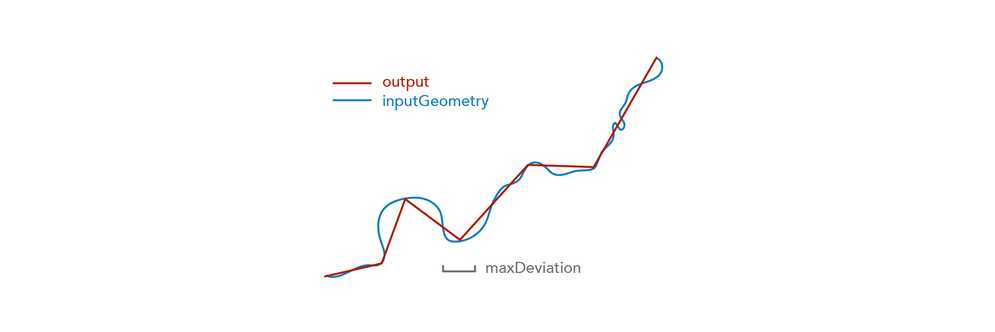##### Example

Returns a generalized version of the input geometry

Use dark colors for code blocksCopy
``````// Removes vertices so segments are no more than 100 meters from the original geometry
Generalize(\$feature, 100, true, 'meters')``````

## Geometry

#### Geometry(inputFeature) -> Geometry

Function bundle: Geometry

Constructs a Geometry object from serialized JSON text or a dictionary. The JSON schema must follow the ArcGIS REST API JSON specification for Geometry objects. This function may also return the Geometry of an input feature.

Feature geometries in the visualization and labeling profiles are generalized according to the view's scale resolution to improve drawing performance. Therefore, using a feature's geometry (i.e. `\$feature`) as input to any geometry function in these contexts will return different results at each scale level. Other profiles, such as popup, provide the full resolution geometry.

Parameter

• inputFeature: Feature | Dictionary | Text - The Feature or JSON from which to construct the geometry object.

Return value: Geometry

##### Examples

Returns the geometry of the feature

Use dark colors for code blocksCopy
``Geometry(\$feature)``

Constructs a point geometry. This can be done with any geometry type.

Use dark colors for code blocksCopy
``````var pointJSON = {"x": -118.15, "y": 33.80, "spatialReference": { "wkid": 3857 } };
Geometry(pointJSON);``````

## Intersection

#### Intersection(geometry1, geometry2) -> Geometry

Since version 1.3

Function bundle: Geometry

Constructs the set-theoretic intersection between two geometries and returns a new geometry.

Feature geometries in the visualization and labeling profiles are generalized according to the view's scale resolution to improve drawing performance. Therefore, using a feature's geometry (i.e. `\$feature`) as input to any geometry function in these contexts will return different results at each scale level. Other profiles, such as popup, provide the full resolution geometry.

Parameters

• geometry1: Geometry | Feature - The geometry to intersect with `geometry2`.
• geometry2: Geometry | Feature - The geometry to intersect with `geometry1`.

Return value: Geometry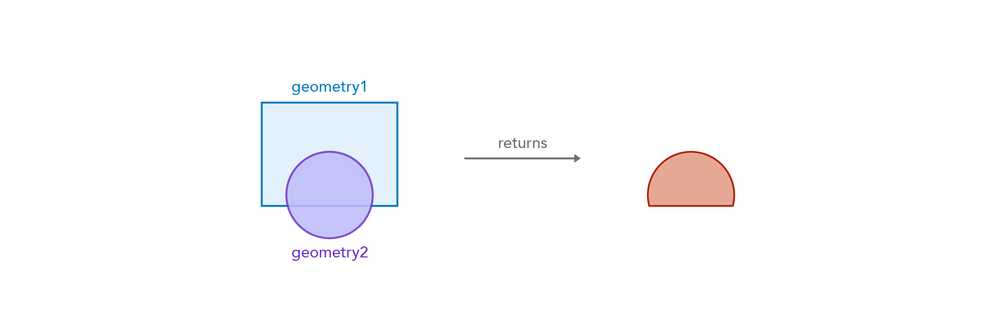##### Example

Returns the area common to both polygons

Use dark colors for code blocksCopy
``````var geom2 = Polygon({ ... });
Area(Intersection(\$feature, geom2), 'square-miles');``````

## Intersects

#### Intersects(geometry1, geometry2) -> Boolean

Since version 1.3

Function bundle: Geometry

Indicates if one geometry intersects another geometry. In the graphic below, the red highlight indicates the scenarios where the function will return `true`.

Feature geometries in the visualization and labeling profiles are generalized according to the view's scale resolution to improve drawing performance. Therefore, using a feature's geometry (i.e. `\$feature`) as input to any geometry function in these contexts will return different results at each scale level. Other profiles, such as popup, provide the full resolution geometry.

Parameters

• geometry1: Geometry | Feature - The geometry that is tested for the intersects relationship to `geometry2`.
• geometry2: Geometry | Feature - The geometry being intersected.

Return value: Boolean##### Example

Returns true if the geometries intersect

Use dark colors for code blocksCopy
``````var geom2 = Polygon({ ... });
Intersects(\$feature, geom2);``````

## IsSelfIntersecting

#### IsSelfIntersecting(inputGeometry) -> Boolean

Since version 1.8

Function bundle: Geometry

Indicates whether the input geometry has rings, paths, or points that intersect or cross other parts of the geometry. For example, a single polyline feature whose paths intersect each other or a polygon with rings that self intersect would return `true`.

Parameter

• inputGeometry: Geometry | Feature - The polygon, polyline, or multipoint geometry to test for the self intersection.

Return value: Boolean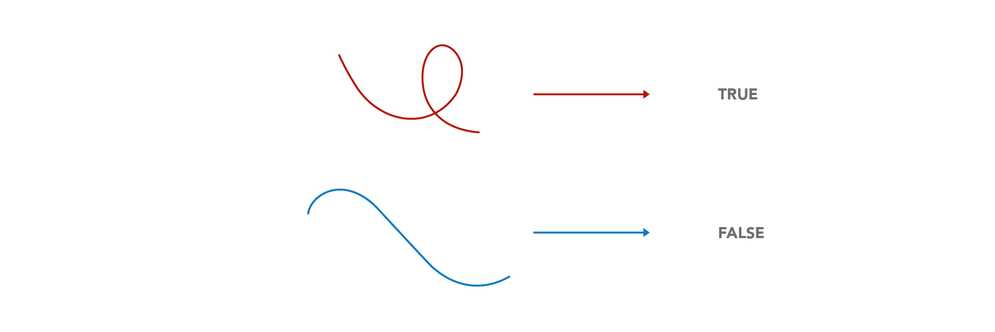##### Example

Returns true if the polyline's paths intersect each other

Use dark colors for code blocksCopy
``````var polyline = Polyline({ ... });
IsSelfIntersecting(polyline);``````

## IsSimple

#### IsSimple(inputGeometry) -> Boolean

Since version 1.11

Function bundle: Geometry

Indicates if the given geometry is topologically simple.

Feature geometries in the visualization and labeling profiles are generalized according to the view's scale resolution to improve drawing performance. Therefore, using a feature's geometry (i.e. `\$feature`) as input to any geometry function in these contexts will return different results at each scale level. Other profiles, such as popup, provide the full resolution geometry.

Parameter

Return value: Boolean

##### Example

Returns true if the geometry is topologically simple

Use dark colors for code blocksCopy
``IsSimple(\$feature);``

## Length

#### Length(inputGeometry, unit?) -> Number

Since version 1.7

Function bundle: Geometry

Returns the length of the input geometry or Feature in the given units. This is a planar measurement using Cartesian mathematics.

Feature geometries in the visualization and labeling profiles are generalized according to the view's scale resolution to improve drawing performance. Therefore, using a feature's geometry (i.e. `\$feature`) as input to any geometry function in these contexts will return different results at each scale level. Other profiles, such as popup, provide the full resolution geometry.

Parameters

• inputGeometry: Geometry | Feature | Array<Point> - The geometry or geometries for which to calculate the planar length.
• unit (Optional): Text | Number - Measurement unit of the return value. For the visualization, labeling, and popup profiles, the default unit is the map's spatial reference. In other profiles, like field calculation, the default is based on the spatial reference of the data.
Possible values: `feet` | `kilometers` | `miles` | `nautical-miles` | `meters` | `yards`

Return value: Number

##### Example

Returns the planar length of the feature in kilometers

Use dark colors for code blocksCopy
``Length(\$feature, 'kilometers')``

## Length3D

#### Length3D(inputGeometry, unit?) -> Number

Since version 1.14

Function bundle: Geometry

Returns the planar (i.e. Cartesian) length of the input geometry or Feature taking height or Z information into account. The geometry provided to this function must be assigned a projected coordinate system. If the spatial reference does not provide a value for Z units, then the result will be returned in meters. Keep in mind that not all clients (such as the 3.x series of the ArcGIS API for JavaScript) support requesting Z values even when the data contains Z information.

Feature geometries in the visualization and labeling profiles are generalized according to the view's scale resolution to improve drawing performance. Therefore, using a feature's geometry (i.e. `\$feature`) as input to any geometry function in these contexts will return different results at each scale level. Other profiles, such as popup, provide the full resolution geometry.

Parameters

• inputGeometry: Geometry | Feature | Array<Point> - The geometry or Feature for which to calculate the planar length in 3D space.
• unit (Optional): Text | Number - Measurement unit of the return value. For the visualization, labeling, and popup profiles, the default unit is the map's spatial reference. In other profiles, like field calculation, the default is based on the spatial reference of the data.
Possible values: `feet` | `kilometers` | `miles` | `nautical-miles` | `meters` | `yards`

Return value: Number

##### Examples

Returns the 3D planar length of the feature in the unit of the spatial reference of the context executing the expression.

Use dark colors for code blocksCopy
``Length3D(\$feature)``

Returns the 3D planar length of the feature in feet.

Use dark colors for code blocksCopy
``Length3D(\$feature, 'feet')``

## LengthGeodetic

#### LengthGeodetic(inputGeometry, unit?) -> Number

Since version 1.7

Function bundle: Geometry

Returns the geodetic length of the input geometry or Feature in the given units. This is more reliable measurement of length than `Length()` because it takes into account the Earth's curvature. Support is limited to geometries with a Web Mercator (wkid 3857) or a WGS 84 (wkid 4326) spatial reference.

Feature geometries in the visualization and labeling profiles are generalized according to the view's scale resolution to improve drawing performance. Therefore, using a feature's geometry (i.e. `\$feature`) as input to any geometry function in these contexts will return different results at each scale level. Other profiles, such as popup, provide the full resolution geometry.

Parameters

• inputGeometry: Geometry | Feature | Array<Point> - The geometry for which to calculate the geodetic length.
• unit (Optional): Text | Number - Measurement unit of the return value. For the visualization, labeling, and popup profiles, the default unit is the map's spatial reference. In other profiles, like field calculation, the default is based on the spatial reference of the data.
Possible values: `feet` | `kilometers` | `miles` | `nautical-miles` | `meters` | `yards`

Return value: Number

##### Example

Returns the geodetic length of the feature in kilometers

Use dark colors for code blocksCopy
``LengthGeodetic(\$feature, 'kilometers')``

## MultiPartToSinglePart

#### MultiPartToSinglePart(inputGeometry) -> Array<Geometry>

Since version 1.3

Function bundle: Geometry

Converts a multi-part geometry into separate geometries.

Feature geometries in the visualization and labeling profiles are generalized according to the view's scale resolution to improve drawing performance. Therefore, using a feature's geometry (i.e. `\$feature`) as input to any geometry function in these contexts will return different results at each scale level. Other profiles, such as popup, provide the full resolution geometry.

Parameter

• inputGeometry: Geometry | Feature - The multi-part geometry to break into single parts.

Return value: Array<Geometry>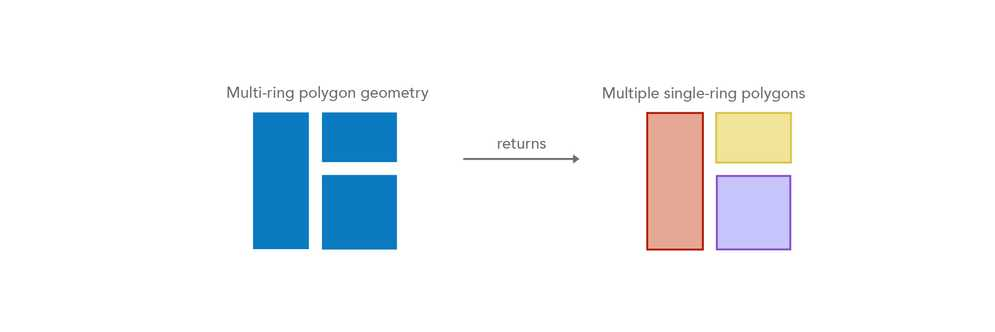##### Example

Returns an array of single-part geometries from a multi-part geometry

Use dark colors for code blocksCopy
``var allParts = MultiPartToSinglePart(\$feature)``

## Multipoint

This function has 3 signatures:

#### Multipoint(geometryDefinition) -> Multipoint

Function bundle: Geometry

Constructs a Multipoint object from a dictionary using only an array of values as coordinates.

Parameter

• geometryDefinition: Dictionary - The properties from which to construct the Multipoint geometry object.

• points: Array<Any> - An array of coordinates representing point locations.

• singlePoint: Array<Number> - An array of numbers representing a single point or coordinate. The first item in the array represents the x-coordinate. The second element represents the y-coordinate. The third element represents the m-coordinate if `hasM` is true and `hasZ` is false. Otherwise, the third element represents the z-coordinate. If `hasZ` and `hasM` are both true, then the third element represents the z-coordinate, and the fourth element represents the m-coordinate.
• hasM: Boolean - Indicates if the geometry has m-values.

• hasZ: Boolean - Indicates if the geometry has z-values.

• spatialReference: Dictionary - The spatial reference of the geometry.

• wkid: Number - The Well-known ID of the geographic or projected coordinate system that defines the reference for which to draw the geometry.

Return value: Multipoint

##### Examples

Creates a Multipoint with only x, y coordinates

Use dark colors for code blocksCopy
``````Multipoint({
points: [
[-97.06138,32.837],
[-97.06133,32.836],
[-97.06124,32.834],
[-97.06127,32.832],
[-97.06138,32.837]
],
spatialReference: { wkid: 3857 }
});``````

Creates a Multipoint with m values

Use dark colors for code blocksCopy
``````Multipoint({
hasM: true,
points: [
[-97.06138,32.837,0],
[-97.06133,32.836,15],
[-97.06124,32.834,30],
[-97.06127,32.832,50],
[-97.06138,32.837,0]
],
spatialReference: { wkid: 3857 }
});``````

Creates a Multipoint with z values

Use dark colors for code blocksCopy
``````Multipoint({
hasZ: true,
points: [
[-97.06138,32.837,1000],
[-97.06133,32.836,1500],
[-97.06124,32.834,1000],
[-97.06127,32.832,500],
[-97.06138,32.837,1000]
],
spatialReference: { wkid: 3857 }
});``````

Creates a Multipoint with z and m values

Use dark colors for code blocksCopy
``````Multipoint({
hasM: true,
hasZ: true,
points: [
[-97.06138,32.837,1000,0],
[-97.06133,32.836,1500,15],
[-97.06124,32.834,1000,30],
[-97.06127,32.832,500,50],
[-97.06138,32.837,1000,0]
],
spatialReference: { wkid: 3857 }
});``````

#### Multipoint(geometryDefinition) -> Multipoint

Function bundle: Geometry

Constructs a Multipoint object from a dictionary using an array of Point objects.

Parameter

• geometryDefinition: Dictionary - The properties from which to construct the Multipoint geometry object.

• points: Array<Point> - An array of point objects.

• hasM: Boolean - Indicates if the geometry has m-values.

• hasZ: Boolean - Indicates if the geometry has z-values.

• spatialReference: Dictionary - The spatial reference of the geometry.

• wkid: Number - The Well-known ID of the geographic or projected coordinate system that defines the reference for which to draw the geometry.

Return value: Multipoint

##### Example

Creates a Multipoint with only x, y coordinates

Use dark colors for code blocksCopy
``````Multipoint({
points: [
Point({ x: -97.06138, y: 32.837, spatialReference: { wkid: 3857 } }),
Point({ x: -97.06133, y: 32.836, spatialReference: { wkid: 3857 } }),
Point({ x: -97.06124, y: 32.834, spatialReference: { wkid: 3857 } }),
Point({ x: -97.06127, y: 32.832, spatialReference: { wkid: 3857 } }),
Point({ x: -97.06138, y: 32.837, spatialReference: { wkid: 3857 } })
],
spatialReference: { wkid: 3857 }
});``````

#### Multipoint(jsonDefinition) -> Multipoint

Function bundle: Geometry

Constructs a Multipoint object from serialized JSON text. The JSON schema must follow the ArcGIS REST API JSON specification for Multipoint objects.

Parameter

• jsonDefinition: Text - The JSON from which to construct the multipoint geometry object.

Return value: Multipoint

##### Example
Use dark colors for code blocksCopy
``````// Creates a Multipoint object
var multipointJSON = '{"points": [[-97.06138,32.837],[-97.06133,32.836],[-97.06124,32.834],[-97.06127,32.832]],"spatialReference" : { "wkid": 3857 }}';
Multipoint(multipointJSON);``````

## NearestCoordinate

#### NearestCoordinate(inputGeometry, searchPoint) -> Dictionary

Since version 1.23

Function bundle: Geometry

Returns the nearest coordinate (not vertex) of the input geometry to the search point. The returned dictionary also includes the shortest planar distance from the search point to the nearest coordinate. If the search point intersects the input geometry, then the search point is returned as the result with a distance of zero.

Feature geometries in the visualization and labeling profiles are generalized according to the view's scale resolution to improve drawing performance. Therefore, using a feature's geometry (i.e. `\$feature`) as input to any geometry function in these contexts will return different results at each scale level. Other profiles, such as popup, provide the full resolution geometry.

Parameters

• inputGeometry: Geometry | Feature - The geometry from which to get the nearest coordinate.
• searchPoint: Point | Feature - The point from which to find the nearest coordinate of the `inputGeometry`.

Return value: Dictionary
Returns a dictionary with the following properties.

• coordinate: Point - The closest coordinate from the `inputGeometry` to the `searchPoint`.
• distance: Number - The planar distance from the closest coordinate to the `searchPoint`. This represents the shortest distance between the `inputGeometry` and the `searchPoint`.
##### Example

Returns the nearest coordinate from the input polygon along with the distance to that coordinate

Use dark colors for code blocksCopy
``````var buildings = FeatureSetByPortalItem(
Portal('https://www.arcgis.com'),
'7b1fb95ab77f40bf8aa09c8b59045449',
0,
['*'],
true
);

var nearestBuilding = First(Intersects(buildings, BufferGeodetic(\$feature, 100, "feet")));

var result = NearestCoordinate(nearestBuilding, \$feature);

return result.distance;

// or

return result.coordinate;``````

## NearestVertex

#### NearestVertex(inputGeometry, searchPoint) -> Dictionary

Since version 1.23

Function bundle: Geometry

Returns the nearest vertex of the input geometry to the search point. The returned dictionary includes the planar distance from the search point to the nearest vertex.

Feature geometries in the visualization and labeling profiles are generalized according to the view's scale resolution to improve drawing performance. Therefore, using a feature's geometry (i.e. `\$feature`) as input to any geometry function in these contexts will return different results at each scale level. Other profiles, such as popup, provide the full resolution geometry.

Parameters

• inputGeometry: Geometry | Feature - The geometry from which to get the nearest vertex.
• searchPoint: Point | Feature - The point from which to find the nearest vertex of the `inputGeometry`.

Return value: Dictionary
Returns a dictionary with the following properties.

• coordinate: Point - The point location representing the nearest vertex from the `inputGeometry` to the `searchPoint`.
• distance: Number - The planar distance from the input geometry's closest vertex to the `searchPoint`.
##### Example

Returns the nearest vertex from the input polygon along with the distance to that vertex

Use dark colors for code blocksCopy
``````var buildings = FeatureSetByPortalItem(
Portal('https://www.arcgis.com'),
'7b1fb95ab77f40bf8aa09c8b59045449',
0,
['*'],
true
);

var nearestBuilding = First(Intersects(buildings, BufferGeodetic(\$feature, 100, "feet")));

var result = NearestVertex(nearestBuilding, \$feature);

return result.distance;

// or

return result.coordinate;``````

## Offset

#### Offset(inputGeometry, offsetDistance, offsetUnit?, joinType?, bevelRatio?, flattenError?) -> Geometry

Since version 1.11

Function bundle: Geometry

Creates a geometry that is a constant planar distance from an input geometry. It is similar to buffering, but produces a one-sided result.

Feature geometries in the visualization and labeling profiles are generalized according to the view's scale resolution to improve drawing performance. Therefore, using a feature's geometry (i.e. `\$feature`) as input to any geometry function in these contexts will return different results at each scale level. Other profiles, such as popup, provide the full resolution geometry.

Parameters

• inputGeometry: Geometry | Feature - The geometry to offset. Point geometries are not supported.
• offsetDistance: Number - The planar distance to offset from the input geometry. If `offsetDistance > 0`, then the offset geometry is constructed to the right of the input geometry, if `offsetDistance = 0`, then there is no change in the geometries, otherwise it is constructed to the left. The direction of the paths or rings of the input geometry determines which side of the geometry is considered right, and which side is considered left. For a simple polygon, the orientation of outer rings is clockwise and for inner rings it is counter clockwise. So the right side of a simple polygon is always its inside.
• offsetUnit (Optional): Text | Number - Measurement unit for `offsetDistance`. Defaults to the units of the input geometry.
Possible values: `feet` | `kilometers` | `miles` | `nautical-miles` | `meters` | `yards`
• joinType (Optional): Text - The join type. Possible values are `round`, `bevel`, `miter`, or `square`.
• bevelRatio (Optional): Number - Applicable when `joinType = 'miter'`; `bevelRatio` is multiplied by the offset distance and the result determines how far a mitered offset intersection can be located before it is beveled.
• flattenError (Optional): Number - Applicable when `joinType = 'round'`; `flattenError` determines the maximum distance of the resulting segments compared to the true circular arc. The algorithm never produces more than around 180 vertices for each round join.

Return value: Geometry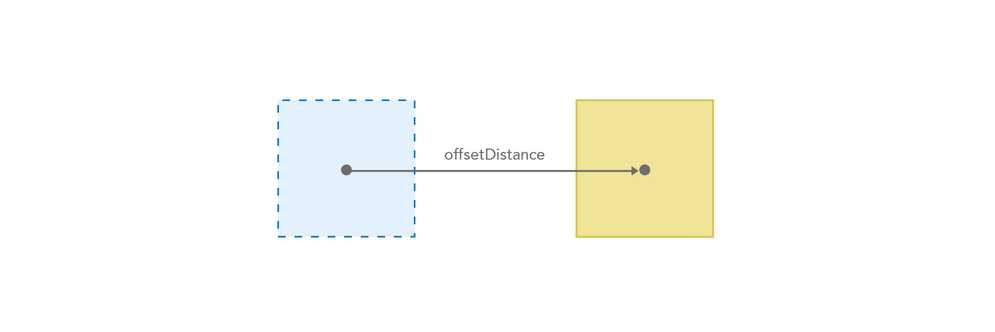##### Example

Returns the offset geometry

Use dark colors for code blocksCopy
``Offset(\$feature, 10, 'meters', 'square');``

## Overlaps

#### Overlaps(geometry1, geometry2) -> Boolean

Since version 1.3

Function bundle: Geometry

Indicates if one geometry overlaps another geometry. In the graphic below, the red highlight indicates the scenarios where the function will return `true`.

Feature geometries in the visualization and labeling profiles are generalized according to the view's scale resolution to improve drawing performance. Therefore, using a feature's geometry (i.e. `\$feature`) as input to any geometry function in these contexts will return different results at each scale level. Other profiles, such as popup, provide the full resolution geometry.

Parameters

• geometry1: Geometry | Feature - The base geometry that is tested for the 'overlaps' relationship with `geometry2`.
• geometry2: Geometry | Feature - The comparison geometry that is tested for the 'overlaps' relationship with `geometry1`.

Return value: Boolean##### Example

Returns true if the geometries overlap

Use dark colors for code blocksCopy
``````var geom2 = Polygon({ ... });
Overlaps(\$feature, geom2);``````

## Point

This function has 2 signatures:

#### Point(geometryDefinition) -> Point

Function bundle: Geometry

Constructs a Point object from a dictionary.

Parameter

• geometryDefinition: Dictionary - The properties from which to construct the Point geometry object.

• x: Number - The x-coordinate (longitude) of the point location.

• y: Number - The y-coordinate (latitude) of the point location.

• m: Number - The m-value (measure) of the point location. If providing an m-value, then you must set `hasM` to `true`.

• z: Number - The z-value (elevation or height) of the point location. If providing a z-value, then you must set `hasZ` to `true`.

• hasM: Boolean - Indicates if the geometry has m-values.

• hasZ: Boolean - Indicates if the geometry has z-values.

• spatialReference: Dictionary - The spatial reference of the geometry.

• wkid: Number - The Well-known ID of the geographic or projected coordinate system that defines the reference for which to draw the geometry.

Return value: Point

##### Examples

Creates a Point with only x, y coordinates

Use dark colors for code blocksCopy
``````Point({
x: -97.06138,
y: 32.837,
spatialReference: { wkid: 3857 }
});``````

Creates a Point with m values

Use dark colors for code blocksCopy
``````Point({
hasM: true,
x: -97.06138,
y: 32.837,
m: 15,
spatialReference: { wkid: 3857 }
});``````

Creates a Point with z values

Use dark colors for code blocksCopy
``````Point({
hasZ: true,
x: -97.06138,
y: 32.837,
z: 1500,
spatialReference: { wkid: 3857 }
});``````

Creates a Point with z and m values

Use dark colors for code blocksCopy
``````Point({
hasM: true,
hasZ: true,
x: -97.06138,
y: 32.837,
z: 1500,
m: 15,
spatialReference: { wkid: 3857 }
});``````

#### Point(jsonDefinition) -> Point

Function bundle: Geometry

Constructs a Point object from serialized JSON text. The JSON schema must follow the ArcGIS REST API JSON specification for Point objects.

Parameter

• jsonDefinition: Text - The JSON from which to construct the point geometry object.

Return value: Point

##### Example
Use dark colors for code blocksCopy
``````// Creates a Point object
var pointJSON = '{ "x": -118.15, "y": 33.80, "spatialReference": { "wkid": 3857 }}';
Point(pointJSON)``````

## Polygon

This function has 3 signatures:

#### Polygon(geometryDefinition) -> Polygon

Function bundle: Geometry

Constructs a Polygon object from a dictionary using only an array of values as coordinates.

Parameter

• geometryDefinition: Dictionary - The properties from which to construct the Polygon geometry object.

• rings: Array<Any> - An array of rings (or shapes) where each ring is an array of vertices.

• ring: Array<Any> - An array of vertices in a ring. All rings should be closed, meaning the first vertex of each ring should always be the same as the last vertex. Vertices should be defined in clockwise order. Rings with vertices defined in counter-clockwise order will result in polygon holes.

• vertex: Array<Number> - An array of numbers representing a single vertex or coordinate in a ring. The first item in the array represents the x-coordinate. The second element represents the y-coordinate. The third element represents the m-coordinate if `hasM` is true and `hasZ` is false. Otherwise, the third element represents the z-coordinate. If `hasZ` and `hasM` are both true, then the third element represents the z-coordinate, and the fourth element represents the m-coordinate.
• hasM: Boolean - Indicates if the geometry has m-values.

• hasZ: Boolean - Indicates if the geometry has z-values.

• spatialReference: Dictionary - The spatial reference of the geometry.

• wkid: Number - The Well-known ID of the geographic or projected coordinate system that defines the reference for which to draw the geometry.

Return value: Polygon

##### Examples

Creates a Polygon with only x, y coordinates

Use dark colors for code blocksCopy
``````// Creates a Polygon with 2 rings
Polygon({
rings: [
[
[-97.06138,32.837],
[-97.06133,32.836],
[-97.06124,32.834],
[-97.06127,32.832],
[-97.06138,32.837]
],
[
[-97.06326,32.759],
[-97.06298,32.755],
[-97.06326,32.759]
]
],
spatialReference: { wkid: 3857 }
});``````

Creates a Polygon with m values

Use dark colors for code blocksCopy
``````// Creates a Polygon with 1 ring
Polygon({
hasM: true,
rings: [
[
[-97.06138,32.837,0],
[-97.06133,32.836,15],
[-97.06124,32.834,30],
[-97.06127,32.832,50],
[-97.06138,32.837,0]
]
],
spatialReference: { wkid: 3857 }
});``````

Creates a Polygon with z values

Use dark colors for code blocksCopy
``````// Creates a Polygon with 1 ring
Polygon({
hasZ: true,
rings: [
[
[-97.06138,32.837,1000],
[-97.06133,32.836,1500],
[-97.06124,32.834,1000],
[-97.06127,32.832,500],
[-97.06138,32.837,1000]
]
],
spatialReference: { wkid: 3857 }
});``````

Creates a Polygon with z and m values

Use dark colors for code blocksCopy
``````// Creates a Polygon with 1 ring
Polygon({
hasM: true,
hasZ: true,
rings: [
[
[-97.06138,32.837,1000,0],
[-97.06133,32.836,1500,15],
[-97.06124,32.834,1000,30],
[-97.06127,32.832,500,50],
[-97.06138,32.837,1000,0]
]
],
spatialReference: { wkid: 3857 }
});``````

#### Polygon(geometryDefinition) -> Polygon

Function bundle: Geometry

Constructs a Polygon object from a dictionary using an array of Points as coordinates.

Parameter

• geometryDefinition: Dictionary - The properties from which to construct the Polygon geometry object.

• rings: Array<Any> - An array of rings (or shapes) where each ring is an array of point objects.

• ring: Array<Point> - An array of points representing a ring. All rings should be closed, meaning the first point of each ring should always be the same as the last point in the ring. Points should be defined in clockwise order. Rings with vertices defined in counter-clockwise order will result in polygon holes.
• hasM: Boolean - Indicates if the geometry has m-values.

• hasZ: Boolean - Indicates if the geometry has z-values.

• spatialReference: Dictionary - The spatial reference of the geometry.

• wkid: Number - The Well-known ID of the geographic or projected coordinate system that defines the reference for which to draw the geometry.

Return value: Polygon

##### Example

Creates a Polygon with only x, y coordinates

Use dark colors for code blocksCopy
``````// Creates a Polygon with 2 rings
Polygon({
rings: [
[
Point({ x: -97.06138, y: 32.837, spatialReference: { wkid: 3857 } }),
Point({ x: -97.06133, y: 32.836, spatialReference: { wkid: 3857 } }),
Point({ x: -97.06124, y: 32.834, spatialReference: { wkid: 3857 } }),
Point({ x: -97.06127, y: 32.832, spatialReference: { wkid: 3857 } }),
Point({ x: -97.06138, y: 32.837, spatialReference: { wkid: 3857 } })
],
[
Point({ x: -97.06326, y: 32.759, spatialReference: { wkid: 3857 } }),
Point({ x: -97.06298, y: 32.755, spatialReference: { wkid: 3857 } }),
Point({ x: -97.06326, y: 32.759, spatialReference: { wkid: 3857 } })
]
],
spatialReference: { wkid: 3857 }
});``````

#### Polygon(jsonDefinition) -> Polygon

Function bundle: Geometry

Constructs a Polygon object from serialized JSON text. The JSON schema must follow the ArcGIS REST API JSON specification for Polygon objects.

Parameter

• jsonDefinition: Text - The JSON from which to construct the polygon geometry object.

Return value: Polygon

##### Example
Use dark colors for code blocksCopy
``````// Creates a Polygon object
var polygonJSON = '{"rings": [[[-97.06138,32.837],[-97.06133,32.836],[-97.06124,32.834],[-97.06127,32.832], [-97.06138,32.837]],[[-97.06326,32.759],[-97.06298,32.755],[-97.06153,32.749], [-97.06326,32.759]]],"spatialReference": { "wkid": 3857 }}';
Polygon(polygonJSON);``````

## Polyline

This function has 3 signatures:

#### Polyline(geometryDefinition) -> Polyline

Function bundle: Geometry

Constructs a Polyline object from a dictionary using only an array of values as coordinates.

Parameter

• geometryDefinition: Dictionary - The properties from which to construct the polyline geometry object.

• paths: Array<Any> - An array of paths (or line segments) where each path is an array of vertices.

• path: Array<Any> - An array of vertices in a line segment, or path.

• vertex: Array<Number> - An array of numbers representing a single vertex or coordinate in a line segment. The first item in the array represents the x-coordinate. The second element represents the y-coordinate. The third element represents the m-coordinate if `hasM` is true and `hasZ` is false. Otherwise, the third element represents the z-coordinate. If `hasZ` and `hasM` are both true, then the third element represents the z-coordinate, and the fourth element represents the m-coordinate.
• hasM: Boolean - Indicates if the geometry has m-values.

• hasZ: Boolean - Indicates if the geometry has z-values.

• spatialReference: Dictionary - The spatial reference of the geometry.

• wkid: Number - The Well-known ID of the geographic or projected coordinate system that defines the reference for which to draw the geometry.

Return value: Polyline

##### Examples

Creates a polyline with only x, y coordinates

Use dark colors for code blocksCopy
``````// Creates a Polyline with 2 paths
Polyline({
paths: [
[
[-97.06138,32.837],
[-97.06133,32.836],
[-97.06124,32.834],
[-97.06127,32.832]
],
[
[-97.06326,32.759],
[-97.06298,32.755]
]
],
spatialReference: { wkid: 3857 }
});``````

Creates a polyline with m values

Use dark colors for code blocksCopy
``````// Creates a Polyline with 1 path
Polyline({
hasM: true,
paths: [
[
[-97.06138,32.837,0],
[-97.06133,32.836,15],
[-97.06124,32.834,30],
[-97.06127,32.832,50]
]
],
spatialReference: { wkid: 3857 }
});``````

Creates a polyline with z values

Use dark colors for code blocksCopy
``````// Creates a Polyline with 1 path
Polyline({
hasZ: true,
paths: [
[
[-97.06138,32.837,1000],
[-97.06133,32.836,1500],
[-97.06124,32.834,1000],
[-97.06127,32.832,500]
]
],
spatialReference: { wkid: 3857 }
});``````

Creates a polyline with z and m values

Use dark colors for code blocksCopy
``````// Creates a Polyline with 1 path
Polyline({
hasM: true,
hasZ: true,
paths: [
[
[-97.06138,32.837,1000,0],
[-97.06133,32.836,1500,15],
[-97.06124,32.834,1000,30],
[-97.06127,32.832,500,50]
]
],
spatialReference: { wkid: 3857 }
});``````

#### Polyline(geometryDefinition) -> Polyline

Function bundle: Geometry

Constructs a Polyline object from a dictionary using an array of Points as coordinates.

Parameter

• geometryDefinition: Dictionary - The properties from which to construct the polyline geometry object.

• paths: Array<Any> - An array of paths (or line segments) where each path is an array of point objects.

• path: Array<Point> - An array of points representing a line segment, or path.
• hasM: Boolean - Indicates if the geometry has m-values.

• hasZ: Boolean - Indicates if the geometry has z-values.

• spatialReference: Dictionary - The spatial reference of the geometry.

• wkid: Number - The Well-known ID of the geographic or projected coordinate system that defines the reference for which to draw the geometry.

Return value: Polyline

##### Example

Creates a polyline with only x, y coordinates

Use dark colors for code blocksCopy
``````// Creates a Polyline with 2 paths
Polyline({
paths: [
[
Point({ x: -97.06138, y: 32.837, spatialReference: { wkid: 3857 } }),
Point({ x: -97.06133, y: 32.836, spatialReference: { wkid: 3857 } }),
Point({ x: -97.06124, y: 32.834, spatialReference: { wkid: 3857 } }),
Point({ x: -97.06127, y: 32.832, spatialReference: { wkid: 3857 } })
],
[
Point({ x: -97.06326, y: 32.759, spatialReference: { wkid: 3857 } }),
Point({ x: -97.06298, y: 32.755, spatialReference: { wkid: 3857 } })
]
],
spatialReference: { wkid: 3857 }
});``````

#### Polyline(jsonDefinition) -> Polyline

Function bundle: Geometry

Constructs a Polyline object from serialized JSON text. The JSON schema must follow the ArcGIS REST API JSON specification for Polyline objects.

Parameter

• jsonDefinition: Text - The JSON text from which to construct the polyline geometry object.

Return value: Polyline

##### Example
Use dark colors for code blocksCopy
``````// Creates a Polyline object
var polylineJSON = '{"paths": [[[-97.06138,32.837],[-97.06133,32.836],[-97.06124,32.834],[-97.06127,32.832]], [[-97.06326,32.759],[-97.06298,32.755]]], "spatialReference": { "wkid": 3857 } }'
Polyline(polylineJSON);``````

## Relate

#### Relate(geometry1, geometry2, relation) -> Boolean

Since version 1.11

Function bundle: Geometry

Indicates if the given DE-9IM relation is `true` for the two geometries.

Feature geometries in the visualization and labeling profiles are generalized according to the view's scale resolution to improve drawing performance. Therefore, using a feature's geometry (i.e. `\$feature`) as input to any geometry function in these contexts will return different results at each scale level. Other profiles, such as popup, provide the full resolution geometry.

Parameters

• geometry1: Geometry | Feature - The first geometry for the relation.
• geometry2: Geometry | Feature - The second geometry for the relation.
• relation: Text - The Dimensionally Extended 9 Intersection Model (DE-9IM) matrix relation (encoded as a text value) to test against the relationship of the two geometries. This text contains the test result of each intersection represented in the DE-9IM matrix. Each result is one character of the text and may be represented as either a number (maximum dimension returned: 0,1,2), a Boolean value (T or F), or a mask character (for ignoring results: '*').

Example: Each of the following DE-9IM text codes are valid for testing whether a polygon geometry completely contains a line geometry: TTTFFTFFT (Boolean), 'T******FF*' (ignore irrelevant intersections), or '102FF*FF*' (dimension form). Each returns the same result.

Return value: Boolean

##### Example

Returns true if the relation of the input geometries matches

Use dark colors for code blocksCopy
``Relate(\$feature, geometry2, 'TTTFFTFFT')``

## RingIsClockwise

#### RingIsClockwise(points) -> Boolean

Since version 1.7

Function bundle: Geometry

Indicates whether the points in a polygon ring are ordered in a clockwise direction.

Parameter

• points: Array<Point> - An array of points in a polygon ring.

Return value: Boolean

##### Example
Use dark colors for code blocksCopy
``````// \$feature is a polygon feature
var polygonRings = Geometry(\$feature).rings;
IIf(RingIsClockwise(polygonRings), 'correct polygon', 'incorrect direction')``````

## Rotate

#### Rotate(inputGeometry, angle, rotationOrigin?) -> Geometry

Since version 1.11

Function bundle: Geometry

Rotates a geometry counterclockwise by the specified number of degrees. Rotation is around the centroid, or a given rotation point.

Feature geometries in the visualization and labeling profiles are generalized according to the view's scale resolution to improve drawing performance. Therefore, using a feature's geometry (i.e. `\$feature`) as input to any geometry function in these contexts will return different results at each scale level. Other profiles, such as popup, provide the full resolution geometry.

Parameters

• inputGeometry: Geometry | Feature - The geometry to rotate.
• angle: Number - The rotation angle in degrees.
• rotationOrigin (Optional): Point - Point to rotate the geometry around. Defaults to the centroid of the geometry.

Return value: Geometry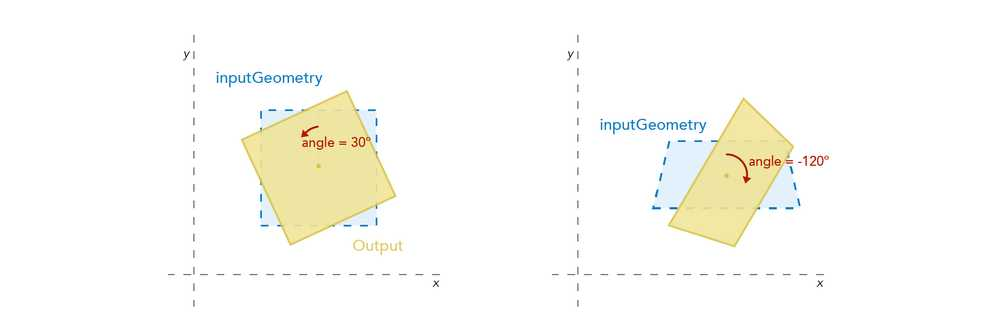##### Example

Returns the input feature rotated about the centroid by 90 degrees

Use dark colors for code blocksCopy
``Rotate(\$feature, 90)``

## SetGeometry

#### SetGeometry(inputFeature, inputGeometry) -> Null

Since version 1.3

Function bundle: Geometry

Sets or replaces a geometry on a user-defined Feature. Note that features referenced as global variables are immutable; their geometries cannot be changed.

Feature geometries in the visualization and labeling profiles are generalized according to the view's scale resolution to improve drawing performance. Therefore, using a feature's geometry (i.e. `\$feature`) as input to any geometry function in these contexts will return different results at each scale level. Other profiles, such as popup, provide the full resolution geometry.

Parameters

• inputFeature: Feature - A feature whose geometry will be updated.
• inputGeometry: Geometry - The geometry to set on the input feature.

Return value: Null

##### Example

Sets a new geometry on the feature

Use dark colors for code blocksCopy
``````var pointFeature = Feature(Point( ... ), 'name', 'buffer centroid');
var mileBuffer = BufferGeodetic(Geometry(pointFeature), 1, 'mile');
SetGeometry(pointFeature, mileBuffer);``````

## Simplify

#### Simplify(inputGeometry) -> Geometry

Since version 1.11

Function bundle: Geometry

Performs the simplify operation on the geometry. This alters the given geometry to make it topologically legal.

Feature geometries in the visualization and labeling profiles are generalized according to the view's scale resolution to improve drawing performance. Therefore, using a feature's geometry (i.e. `\$feature`) as input to any geometry function in these contexts will return different results at each scale level. Other profiles, such as popup, provide the full resolution geometry.

Parameter

Return value: Geometry

##### Example

Returns the simplified geometry of the feature

Use dark colors for code blocksCopy
``Simplify(\$feature);``

## SymmetricDifference

#### SymmetricDifference(leftGeometry, rightGeometry) -> Geometry

Since version 1.3

Function bundle: Geometry

Performs the Symmetric difference operation on the two geometries. The symmetric difference includes the parts of both geometries that are not common with each other.

Feature geometries in the visualization and labeling profiles are generalized according to the view's scale resolution to improve drawing performance. Therefore, using a feature's geometry (i.e. `\$feature`) as input to any geometry function in these contexts will return different results at each scale level. Other profiles, such as popup, provide the full resolution geometry.

Parameters

• leftGeometry: Geometry | Feature - The geometry instance to compare to `rightGeometry` in the XOR operation.
• rightGeometry: Geometry | Feature - The geometry instance to compare to `leftGeometry` in the XOR operation.

Return value: Geometry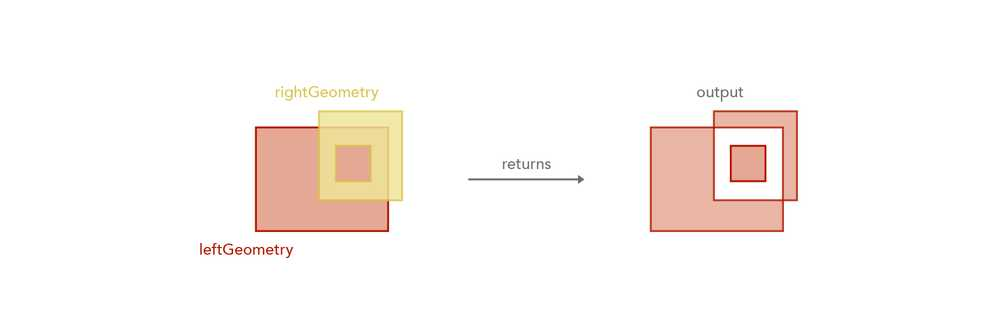##### Example

Returns a polygon representing areas where both inputs do not overlap

Use dark colors for code blocksCopy
``````var geom2 = Polygon({ ... });
SymmetricDifference(\$feature, geom2);``````

## Touches

#### Touches(geometry1, geometry2) -> Boolean

Since version 1.3

Function bundle: Geometry

Indicates if one geometry touches another geometry. In the graphic below, the red highlight indicates the scenarios where the function will return `true`.

Feature geometries in the visualization and labeling profiles are generalized according to the view's scale resolution to improve drawing performance. Therefore, using a feature's geometry (i.e. `\$feature`) as input to any geometry function in these contexts will return different results at each scale level. Other profiles, such as popup, provide the full resolution geometry.

Parameters

• geometry1: Geometry | Feature - The geometry to test the 'touches' relationship with `geometry2`.
• geometry2: Geometry | Feature - The geometry to test the 'touches' relationship with `geometry1`.

Return value: Boolean##### Example

Returns true if the geometries touch

Use dark colors for code blocksCopy
``````var geom2 = Polygon({ ... });
Touches(\$feature, geom2);``````

## Union

This function has 2 signatures:

#### Union(geometries) -> Geometry

Since version 1.3

Function bundle: Geometry

Constructs the set-theoretic union of the geometries or features in an input array and returns a single Geometry. All inputs must have the same geometry type and share the same spatial reference.

Be aware that using `\$feature` as input to this function will yield results only as precise as the view's scale resolution. Therefore values returned from expressions using this function may change after zooming between scales.

Parameter

• geometries: Array<Geometry> | Array<Feature> - An array of geometries or an array of features to union into a single geometry. This can be any number of geometries.

Return value: Geometry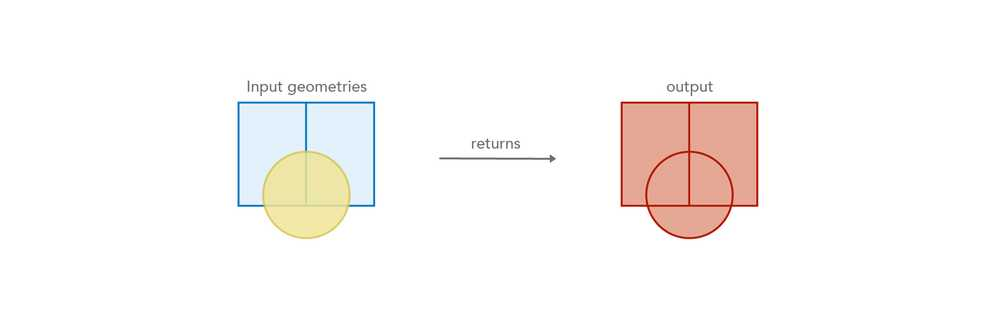##### Example
Use dark colors for code blocksCopy
``````var geom2 = Polygon({ ... });
Union([ \$feature, geom2 ]);``````

#### Union(geometry1, [geometry2, ..., geometryN]?) -> Geometry

Since version 1.3

Function bundle: Geometry

Constructs the set-theoretic union of a list of geometries and returns a single Geometry. All inputs must have the same geometry type and share the same spatial reference.

Be aware that using `\$feature` as input to this function will yield results only as precise as the view's scale resolution. Therefore values returned from expressions using this function may change after zooming between scales.

Parameters

• geometry1: Geometry | Feature - A geometry to union into a single geometry with the other geometries.
• [geometry2, ..., geometryN] (Optional): Geometry | Feature - An ongoing list of geometries or features to union into a single geometry. This can be any number of geometries.

Return value: Geometry##### Example
Use dark colors for code blocksCopy
``````var geom2 = Polygon({ ... });
var geom3 = Polygon({ ... });
var geom4 = Polygon({ ... });
Union(Geometry(\$feature), geom2, geom3, geom4);``````

## Within

#### Within(innerGeometry, outerGeometry) -> Boolean

Since version 1.7

Function bundle: Geometry

Indicates if one geometry is within another geometry. In the graphic below, the red highlight indicates the scenarios where the function will return `true`.

Feature geometries in the visualization and labeling profiles are generalized according to the view's scale resolution to improve drawing performance. Therefore, using a feature's geometry (i.e. `\$feature`) as input to any geometry function in these contexts will return different results at each scale level. Other profiles, such as popup, provide the full resolution geometry.

Parameters

• innerGeometry: Geometry | Feature - The base geometry that is tested for the 'within' relationship to `outerGeometry`.
• outerGeometry: Geometry | Feature - The comparison geometry that is tested for the 'contains' relationship to `innerGeometry`.

Return value: Boolean##### Example

Returns true if the feature is within the given polygon

Use dark colors for code blocksCopy
``````var outerGeom = Polygon({ ... });
Within(\$feature, outerGeom);``````